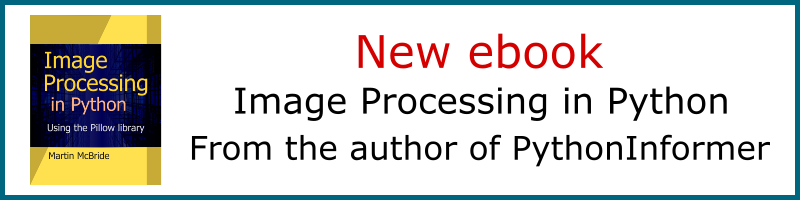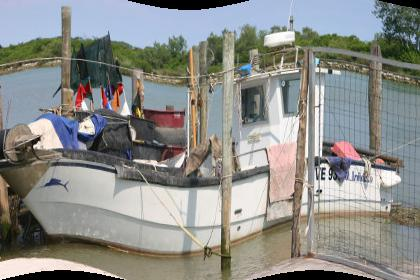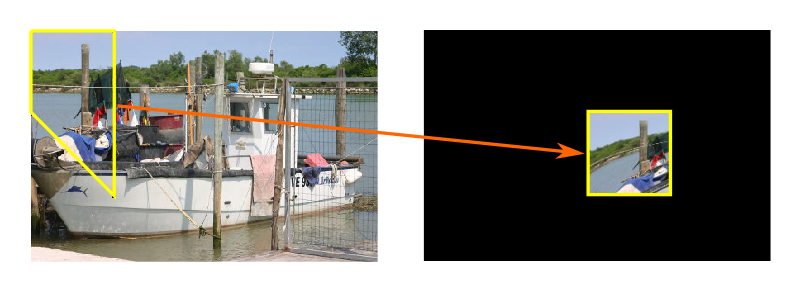# Image deforming recipes in Pillow

Martin McBride, 2021-07-24
Tags image processing recipes
Categories pillowThe ImageOps deform function can warp and deform images in various way.

## Deforming images

You can use the ImageOps deform function to apply general deformations to an image. Typical deformations include:

• Barrel distortion. This happens when an image appears more magnified at its centre than its edges. It is sometimes called a fisheye effect.
• Pincushion distortion, which is the opposite of barrel distortion.
• Perspective distortion. For example, if you take a photograph looking up at a tall building, the building will appear narrower at the top due to perspective.

You can use the deform function to correct for these types of distortion in a photograph. You can also use it to add these types of distortion to a photograph for artistic effect. There are many other types of deformation you can add too.

In this article we will look at how to add this simple wave deformation to an image:Before we get to that, we will look at a simpler case to better understand how the function works.

### How deform works

Deformation takes an existing image (the source image) and creates a new image of the same size and mode (the target image).

The deformation is controlled by a mesh:

• The mesh defines one or more rectangular regions on the target image. Each rectangle is aligned with the x and y axes of the image.
• For each target region, the mesh defines a quadrilateral region in the source image.
• deform copies each region from the source to the target. The source region is deformed to fit the target rectangle.

Here is a simple deformation that maps one region of the source image onto one region of the destination image:Here the non-rectangular source region is translated to a new position in the target image and also squeezed into a square shape. Since the source quad is a different shape to the target rectangle, the image data has to be distorted to make it fit. This is an important aspect of how deformation works - the source image is never cropped, it is stretched or squeezed to fit it into the target rectangle.

It is possible to scale, rotate, mirror, skew, or deform the region, depending on the shape of the source quadrilateral and the order of the vertices.

This example shows a single region. The code is described below. In most cases, you will divide the image into many regions to apply a deformation to the whole image.

### getmesh

The deform function needs to know how to map the target image onto the required regions of the source image. To do that, it uses a deformer object.

The deformer object is any object implement a getmesh function, which:

• Accepts the image as a parameter.
• Returns a list of mappings.

Here is a simple deformer that maps the single region we saw above:

class SingleDeformer:

def getmesh(self, img):
#Map a target rectangle onto a source quad
return [(
# target rectangle
(200, 100, 300, 200),
(0, 0, 0, 100, 100, 200, 100, 0)
)]


A mapping takes the form:

((200, 100, 300, 200), (0, 0, 0, 100, 100, 200, 100, 0))


This mapping contains:

• The target rectangle, defined by the two points (200, 100) and (300, 200).
• The source quad, represented by the four points (0, 0), (0, 100), (100, 200), and (100, 0).

Since the target is a rectangle, aligned to the axes, we can specify it using just two points, the top left and the bottom right. This completely defines the rectangle. However, for the source quad, we need to specify all four corners:

• The top left (ie the corner that maps on to the top left of the target rectangle).
• The bottom left.
• The bottom right.
• The top right.

getmesh is required to return a list of mappings. There is only one mapping in this simple case, so we must return a single list with just one mapping:

[((200, 100, 300, 200), (0, 0, 0, 100, 100, 200, 100, 0))]


Here is how we use this deformer on an image:

image = Image.open('boat-small.jpg')
result_image = ImageOps.deform(image, SingleDeformer())
result_image.save('imageops-deform.jpg')


The result of this mapping was shown in the image above.

### A wave transform

Now we will take a look at the wave-transform from the beginning of this section. This requires us to divide the image up into lots of small regions, and map each one separately. This diagram shows the regions we will use:The right-hand side shows the target mesh. It is a grid of 20 by 20 pixel squares.

The left-hand side shows the source quads. Each square of the target is taken from a parallelogram-shaped area of the source image, that has been displaced and sheared relative to the target grid. The overall effect of this is to make a wavey image.

Here is the wave deformer:

class WaveDeformer:

def transform(self, x, y):
y = y + 10*math.sin(x/40)
return x, y

def transform_rectangle(self, x0, y0, x1, y1):
return (*self.transform(x0, y0),
*self.transform(x0, y1),
*self.transform(x1, y1),
*self.transform(x1, y0),
)

def getmesh(self, img):
self.w, self.h = img.size
gridspace = 20

target_grid = []
for x in range(0, self.w, gridspace):
for y in range(0, self.h, gridspace):
target_grid.append((x, y, x + gridspace, y + gridspace))

source_grid = [self.transform_rectangle(*rect) for rect in target_grid]

return [t for t in zip(target_grid, source_grid)]


There are lots of squares in the mesh, so we will create them programmatically inside getmesh. First, the target mesh:

        target_grid = []
for x in range(0, self.w, gridspace):
for y in range(0, self.h, gridspace):
target_grid.append((x, y, x + gridspace, y + gridspace))


This simply creates a set of squares, of size gridspace by gridspace, that completely cover the image.

Next we create an corresponding set of source quads:

        source_grid = [self.transform_rectangle(*rect) for rect in target_grid]


transform_rectangle is called once for each square (x0, y0, x1, y1). It expands this square into a quad with corners (x0, y0), (x0, y1), (x1, y1), and (x1, y0). It then calls transform to transform the position of each corner.

It is the transform function that controls what happens to the image. The function leaves the x coordinate unchanged but displaces the y component by a function related to the sine of x. This causes the image to be displaced vertically as you move along the image in the horizontal direction.

### Other deformations

The WaveDeformer class provides a general method of creating a set of target squares and corresponding source quads.

You can create a variety of other deformations simply by altering the transform method.

If you found this article useful, you might be interested in the book Image Processing in Python or other books by the same author.

#### Popular tags

2d arrays abstract data type alignment and angle animation arc array arrays bar chart bar style behavioural pattern bezier curve built-in function callable object chain circle classes clipping close closure cmyk colour combinations comparison operator comprehension context context manager conversion count creational pattern data science data types decorator design pattern device space dictionary drawing duck typing efficiency ellipse else encryption enumerate fill filter font font style for loop function function composition function plot functools game development generativepy tutorial generator geometry gif global variable gradient greyscale higher order function hsl html image image processing imagesurface immutable object in operator index inner function input installing iter iterable iterator itertools join l system lambda function len lerp line line plot line style linear gradient linspace list list comprehension logical operator lru_cache magic method mandelbrot mandelbrot set map marker style matplotlib monad mutability named parameter numeric python numpy object open operator optimisation optional parameter or pandas partial application path pattern permutations pie chart polygon positional parameter print pure function python standard library radial gradient range recipes rectangle recursion reduce regular polygon repeat rgb rotation roundrect scaling scatter plot scipy sector segment sequence setup shape singleton slice slicing sound spirograph sprite square str stream string stroke structural pattern subpath symmetric encryption template text text metrics tinkerbell fractal transform translation transparency triangle truthy value tuple turtle unpacking user space vectorisation webserver website while loop zip